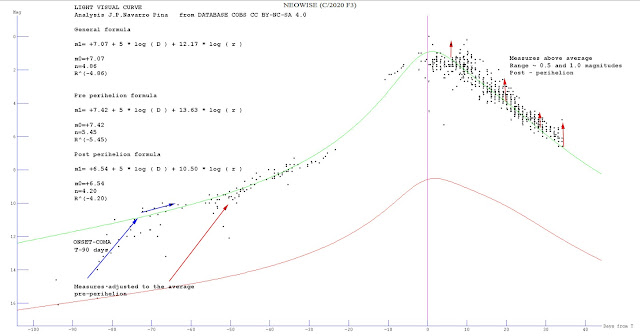## domingo, 2 de diciembre de 2018

### PRELIMINARY ANALYSIS OF THE CURVE OF VISUAL LIGHT AND CCD OF THE COMET 46 P / WIRTANEN

ABSTRACT A preliminary analysis of the visual light curve and ccd of Comet 46P / Wirtanen with 990 visual measurements and ccd obtained from the sources MPC / IAU, COBS database, and observadorescometas yahoogroups.com (Navarro Pina Administrator) is made, being the largest curve of light until the moment of this comet, are determined, the photometric parameters of the comet, absolute magnitude (m0), and rate of increase in brightness or activity index (n), as well as an analysis and revision of the physical parameters, diameter of the nucleus, mass, rate of water production, also the author, studies the correlation between magnitudes ccd (mccd) and visual (m1), finding an increase in time with a difference of 100 days of 4.4 magnitudes, and obtaining the equations [ d (m1-mccd) T-20 = 6.1 mag. ] and [ d (m1-mccd) T-20 = 1.7 mag.] 1. INTRODUCTION AND RESUME Studying the resulting light curve at only 20 days for perihelion, we found, with 990 visual observations and ccd collected from the MPC database and the COBS source, I have also been very helpful in visual observations of the comet. M.Goiato (Brazil) is a regular collaborator of the yahoogroups.com obserdorescometas page, and of S. Yoshida (Japan), a regular collaborator who sends me measurements of comets, thanks to the two astronomers for their collaboration. First of all I decided to perform a joint analysis of the data without differentiating ccd magnitudes and visual magnitudes to obtain the raw data of the photometric parameters being the resulting equation: 1.0 m1 = 13.7 + 5log (d) + 15.7log (R) Since d is the distance comet earth and R the distance comet sun, this equation is based on the classic formula for studying the brightness of comets: 1.1 m1 = m0 + 5log (d) + n log (R) being m0 the absolute magnitude and n the rate of increase in brightness of the comet, the latter contains valuable physical information of the comet. The results indicate an m0 = 13.7 and an index n = 6.3, n indicates that the nucleus of the comet at T-20 days for perihelion contains a high rate of volatile compounds sublimated from the nucleus. Applying the formulas of C.Morris and S.Hanner: 1.2 log Q (H2O) = -n log (R) 1.3 log Q (C2) = -n log (R) **Work in project**

### Update Light Visual Curve C/2020 F3 NEOWISE

New update of the visual light curve of comet NEOWISE, based on 798 observations of the comet, from the COBS database, my analysis includes ...